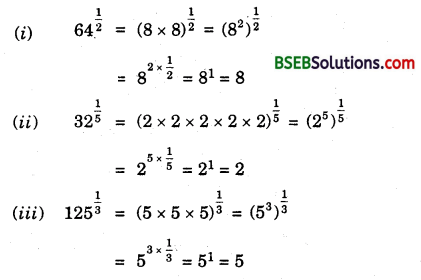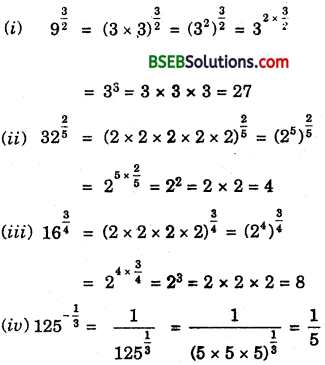# Bihar Board Class 9th Maths Solutions Chapter 1 Number Systems Ex 1.6

Bihar Board Class 9th Maths Solutions Chapter 1 Number Systems Ex 1.6 Textbook Questions and Answers.

## BSEB Bihar Board Class 9th Maths Solutions Chapter 1 Number Systems Ex 1.6Question 1.
Find : (i) $$64^{\frac{1}{2}}$$
(ii) $$32^{\frac{1}{5}}$$
(iii) $$125^{\frac{1}{3}}$$
Solution:Question 2.
Find : (i) $$9^{\frac{3}{2}}$$
(ii) $$32^{\frac{2}{5}}$$
(iii) $$16^{\frac{3}{4}}$$
(iv) $$125^{\frac{-1}{3}}$$
Solution:Question 3.
Simplify : (i) $$2^{\frac{2}{3}} \cdot 2^{\frac{1}{5}}$$
(ii) $$\left(\frac{1}{3^{3}}\right)^{7}$$
(iii) $$\frac{11^{\frac{1}{2}}}{11^{\frac{1}{4}}}$$
(iv) $$7^{\frac{1}{2}} \cdot 8^{\frac{1}{2}}$$
Solution: